Vectors and conics

This free course is available to start right now. Review the full course description and key learning outcomes and create an account and enrol if you want a free statement of participation.

Free course

3.2 Post-audio exercises

Example 42

Let u and v be the position vectors (6, 8) and (−12, 5), respectively.

• (a) Sketch u and v on a single diagram. On the same diagram, sketch the projection of u onto v, and the projection of v onto u.

• (b) Determine the angle between u and v.

• (c) Determine the projection of u onto v, and the projection of v onto u.

• (a)

•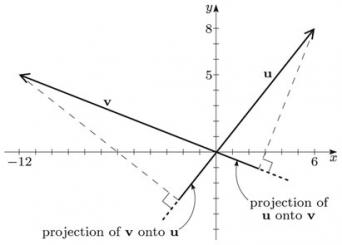• (b) Let θ denote the angle between u and v. Now

•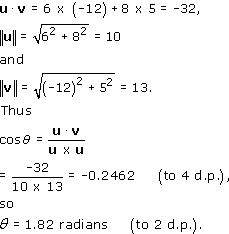• (c) The projection of u onto v is

•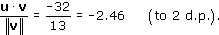• The projection of v onto u is

•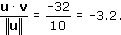Example 43

Determine the angle between the vectors u = (3, 4, 5) and v = (1, 0, −1) in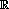3.

Let θ denote the angle between u and v. Now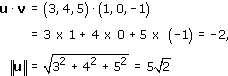and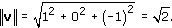Thus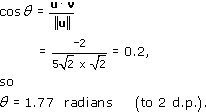Example 44

Find a vector of length 2 that is perpendicular to both of the vectors a = (2, 1, 0) and b = (1, 0, −1).

Let the vector we want be denoted by

v = (x,y,z),

for some real numbers x, y and z.

Since ‖v‖ = 2,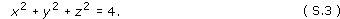Since v is perpendicular to a,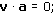thus

2x + y = 0,

that is,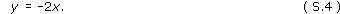Since v is perpendicular to b,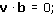thus

x − z = 0,

that is,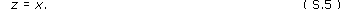Substituting the expressions for y in equation (S.4) and for z in equation (S.5) into equation (S.3), we obtain

x2 + (−2x)2 + x2 = 4;

thus

6x2 = 4,

so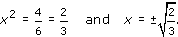It follows that the two possible vectors that satisfy the given conditions are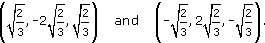M208_1